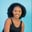Related Tags

numpy
python

# How to find the addition of a 2D array in PythonMaria Elijah

## Overview

The Python library, NumPy, has a method called add(), which can be used to find the addition of two arrays element-wise.

### The numpy.add() method

The numpy.add() method returns the addition of two input arrays. It does it element by element.

Note: A two-dimensional (2D) array can be created using a list of lists in Python.

### Syntax

numpy.add(arr1,arr2, where=True, dtype=None, out=None)


### Parameters

• arr1: This is an array that represents input data.
• arr2: This is an array that represents input data.
• where: This is an optional parameter. The output array will be set to the ufunc result at locations where the condition is True. Otherwise, the output array will keep its original value.
• dtype: This is an optional parameter. It represents the return type of the array.
• out: This is an optional parameter. It represents the alternative output array in which the result is to be placed.

### Return value

The numpy.add() method returns the addition of the given input arrays.

Note: If the out parameter is specified, it returns an array reference to out.

### Example

The following code shows how to find the addition of the two-dimensional (2D) arrays using the numpy.add() method.

# import numpy
import numpy as np
# create an arr
arr1 = ([24,8,3,-4],[2,9,5,-7])
arr2 = ([5,7,2,1],[10,3,-6,9])
# compute the addition of the arrays

print(result)

### Explanation

• Line 2: We import the numpy library.
• Lines 4–5: We create two 2D arrays called arr1 and arr2.
• Line 7: We use the np.add() to compute the addition of the two arrays.
• Line 11: We display the result.

RELATED TAGS

numpy
python

CONTRIBUTORMaria Elijah
RELATED COURSES

View all Courses

Keep Exploring

Learn in-demand tech skills in half the time